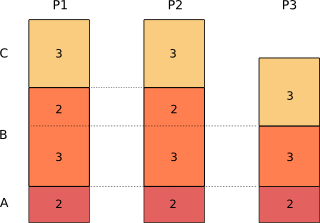## Using procrank to measure memory usage on embedded Linux

One of the fundamental questions programmers ask (or at least, they should) is: how much memory is my program using? It may be a simple question, but with a virtual memory operating system like Linux the answer is quite complex. The numbers given by top and ps don't really add up. Procrank is a tool commonly used by Android platform developers to give more accurate answers, but there is no reason why it can't be more widely used in other Linux based operating systems and it is especially useful for embedded Linux.

# The code

You can get the code from Github: https://github.com/csimmonds/procrank_linux.git

# The theory

The two most common metrics for the memory usage of a process are the virtual set size, Vss, and the resident set size, Rss: you will see see these numbers in ps and top.

Vss, also called VIRT and VSZ is the total amount of virtual memory of the process has mapped, regardless of whether it has been committed to physical memory

Rss, also called RES and RSS, is the amount of physical memory being mapped

The Vss is plainly an overestimate because applications commonly allocate memory they never use. Rss is a better measure, but still an overestimate because it does not take into account pages of memory that are shared between processes. For example, there is only one copy of the C library resident in memory because it is shared between all the processes that link with it yet Rss accounts for it multiple times.

Some years ago, Matt Mackall looked at the problem and added two new metrics called the Unique Set Size, Uss, and the Proportional Set Size, Pss, and modified the kernel to expose the information needed to calculate them, which you will find in /proc/[PID]/smaps.

Uss is unique set size, which is the amount of memory that is private to the process and is not shared with any other

Pss is the proportional set size, which is the amount of memory shared with other processes, divided by the number of processes sharing each page

To over simplify slightly, the diagram below shows three processes and the pages each has mapped into its virtual address space. The pages have been marked as being of type A, B or C where:

• A = private memory that is mapped to physical pages of RAM. This would include the parts of the stack and heap that are being actively used
• B = shared memory that is mapped and is shared by one or more other processes, e.g. code in shared libraries
• C = memory that has been allocated but never touched

And so for each process:

• Vss = A + B + C
• Rss = A + B
• Uss = A
• Pss = A + B/n where n is the number of processes sharingCalculating the Pss for the three processes gives:

Pss(1) = 2 + 3/3 + 2/2 = 4
Pss(2) = 2 + 3/3 + 2/2 = 4
Pss(3) = 2 + 3/3 = 3
Sum(Pss) = 11 = total of pages in use

As you can see, Pss gives an accurate measure of the memory a process is using, taking into account sharing between processes. The total amount of memory in use by all processes is the sum or their Pss.

The Uss is also useful because it shows the pages that are unique. You can think of it as the price you would pay in memory if you forked that process to create a copy.

There is a readily available program that shows Uss and Pss called smem, developed by Matt Mackall. The only problem with it is that it requires a Python run-time environment, which is not often available on an embedded Linux device. The Android developers encountered this problem and they wrote procrank as a command-line tool written in C, using the Android BIONIC C library. I have taken that code, made a few minor changes and added a Makefile so it will compile on most GNU/Linux environments, including cross compiling for embedded use.